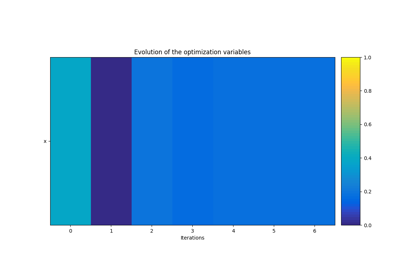gemseo / algos

# opt_result module¶

Optimization result.

class gemseo.algos.opt_result.OptimizationResult(x_0=None, x_opt=None, f_opt=None, status=None, optimizer_name=None, message=None, n_obj_call=None, n_grad_call=None, n_constr_call=None, is_feasible=False, optimum_index=None, constraints_values=None, constraints_grad=None)[source]

Bases: object

The result of an optimization.

Parameters:
• x_0 (ndarray | None) –

• x_opt (ndarray | None) –

• f_opt (ndarray | None) –

• status (int | None) –

• optimizer_name (str | None) –

• message (str | None) –

• n_obj_call (int | None) –

• n_grad_call (int | None) –

• n_constr_call (int | None) –

• is_feasible (bool) –

By default it is set to False.

• optimum_index (int | None) –

• constraints_values (Mapping[str, ndarray] | None) –

• constraints_grad (Mapping[str, ndarray] | None) –

classmethod from_dict(dict_)[source]

Create an optimization result from a dictionary.

Parameters:
• dict – The dictionary representation of the optimization result. The keys are the names of the optimization result fields, except for the constraint values and gradients. The value associated with the key "constr:y" will be stored in result.constraints_values["y"] while the value associated with the key "constr_grad:y" will be stored in result.constraints_grad["y"].

• dict_ (Mapping[str, Union[str, int, bool, ndarray]]) –

Returns:

An optimization result.

Return type:

OptimizationResult

to_dict()[source]

Convert the optimization result to a dictionary.

The keys are the names of the optimization result fields, except for the constraint values and gradients. The key "constr:y" maps to result.constraints_values["y"] while "constr_grad:y" maps to result.constraints_grad["y"].

Returns:

A dictionary representation of the optimization result.

Return type:
constraints_grad: Mapping[str, ndarray] | None = None

The values of the gradients of the constraints at the optimum.

constraints_values: Mapping[str, ndarray] | None = None

The values of the constraints at the optimum.

f_opt: ndarray | None = None

The value of the objective function at the optimum.

is_feasible: bool = False

Whether the solution is feasible.

message: str | None = None

The message returned by the optimizer.

n_constr_call: int | None = None

The number of calls to the constraints function.

n_grad_call: int | None = None

The number of calls to the gradient function.

n_obj_call: int | None = None

The number of calls to the objective function.

optimizer_name: str | None = None

The name of the optimizer.

optimum_index: int | None = None

The zero-based position of the optimum in the optimization history.

status: int | None = None

The status of the optimization.

x_0: ndarray | None = None

The initial values of the design variables.

x_opt: ndarray | None = None

The optimal values of the design variables, called the optimum.

## Examples using OptimizationResult¶Create a DOE Scenario

Create a DOE ScenarioCreate an MDO Scenario

Create an MDO ScenarioAnalytical test case # 2

Analytical test case # 2